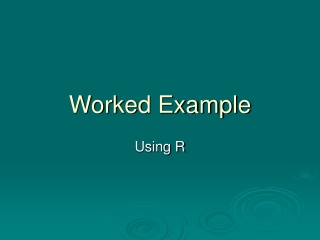DownloadDownload PresentationWorked Example

# Worked Example

Download Presentation## Worked Example

- - - - - - - - - - - - - - - - - - - - - - - - - - - E N D - - - - - - - - - - - - - - - - - - - - - - - - - - -
##### Presentation Transcript

1. Worked Example Using R

2. > plot(y~x)

3. >plot(epsilon1~x) This is a plot of residuals against the exploratory variable, x

4. >plot(epsilon1~yhat) This is a plot of residuals against the fitted values, yhat.

5. Both graphs show the same thing … the residuals are following a random pattern. Note: Since the equation is approximately y=x, both graphs are extremely similar in this case.

6. Model Diagnostics: Residuals and Influence

7. Consider again the problem of fitting the model yi = f(xi) + εi i = 1,……….n Assume again a single continuous response variable y. The explanatory variable x may be either a single variable, or a vector of variables. How do we assess the quality of a given fit f?

8. While summary statistics are helpful, they are not sufficient. Good diagnostics are typically based on case analysis, i.e. an examination of each observation in turn in relation to the fitting procedure. This leads to an examination of residuals and influence.

9. Residuals The residuals should be thought of as what is left of the values of the response variable after the fit has been subtracted. Ideally they should show no further dependence (especially no further location dependence) on x.

10. In general this should be investigated graphically by plotting residuals against the explanatory variable(s) x. For linear models, we frequently compromise by plotting residuals against fitted values.

11. In particular the residuals provide information about: *whether the best relation has been fitted *the relative merits of different fits *mild, but non-random, departures from the hypothesised fit *the magnitude of the residual variation

12. *the identification of outliers *possible further dependence on x, other than through location, of the conditional distribution of y given x - in particular heterogeneity of spread of the residuals.

13. Example:Anscombe’s Artificial Data The R data frame anscombe is made available by > data(anscombe) This contains 4 artificial datasets, each of 11 observations of a continuous response variable y and a continuous explanatory variable x. The data are now plotted along with the result of the least squares linear model to the corresponding dataset.

14. All the usual summary statistics related to the classical analyses of the fitted models are identical across the 4 datasets. This includes the coefficients a and b and their standard errors and confidence intervals, together with the residual standard errors and correlation coefficients. ^ ^

15. Consideration of the residuals shows that very different judgements should be made about the appropriateness of the fitted model to each of the 4 cases. A full discussion is given by Weisberg (1985, pp107,108).

16. Influence Influence measures the extent to which a fit is affected by individual observations. A possible formal definition is the following: the influence of any observation is a measure of the difference between the fit and the fit which would be obtained if that observation were omitted.

17. Obviously observations with large influences require more careful checking. Especially for linear models, influence is often measured by Cook's distance.

18. Cook’s Distance Formula

19. As a rule of thumb, observations for which Di > 1 make a noticeable difference to the parameter estimates, and should be examined carefully for the appropriateness of their use in fitting the model. Clearly an observation with a large residual also has a large influence. However, an observation with an unusual value of its explanatory variable(s) can pull a fit towards it and have a large influence though a small residual.

20. Example: Anscombe's third data set. The last graph produced by the plot function shows that the observation number 3 has an unusually large value of Cook's distance D3 = 1.39. >plot(model3) produces:

21. We now refit the data omitting this observation. >x5=x3[-3] >y5=y3[-3] >model5=lm(y5~x5)